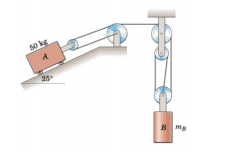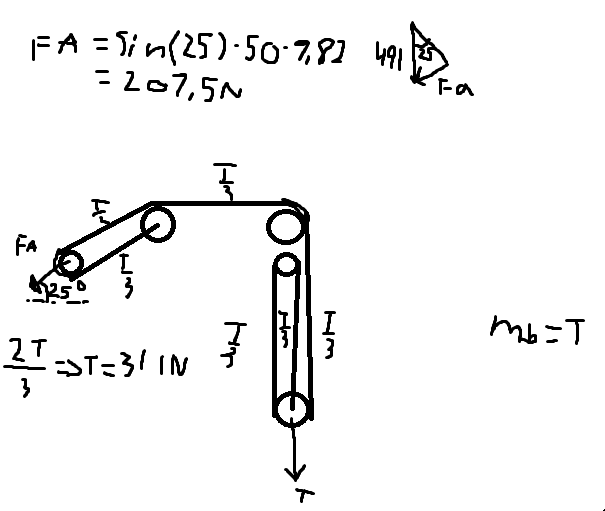# Pulley system -- Calculate the mass that balances this pulley system

FFTB
Homework Statement:
Calculate the mass of mB that makes the system to be in equilibrium. Neglect the possible friction
Relevant Equations:
Sin cos tanI've been stuck at this pulley system for a while.
I've calculated the force of which A pulls => FA = sin25*50*9.82 = 208.5 N
But I get stuck on the free body diagram.
Can someone help and explain the freebody diagram#### Attachments

Mentor
Let T be the tension in the rope. Does it vary along the rope? What is the tension force on mass A? On mass B?